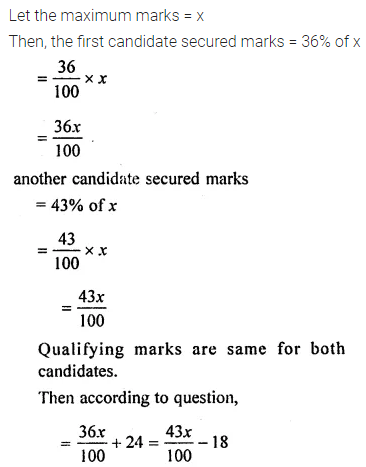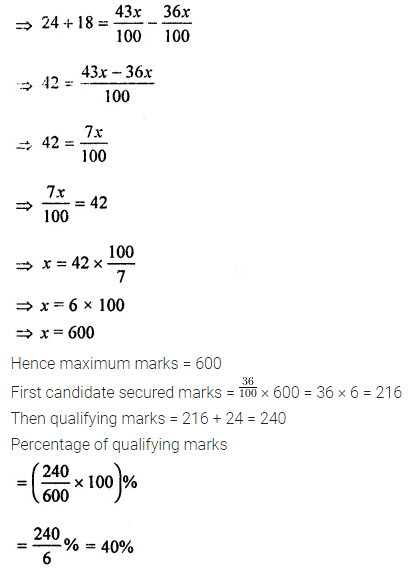## ML Aggarwal Class 8 Solutions for ICSE Maths Chapter 7 Percentage Ex 7.1

Question 1.
Express the following percentages as fractions:
(i) 356%
(ii) $$2 \frac{1}{2}$$%
(iii) $$16 \frac{2}{2}$$%
Solution: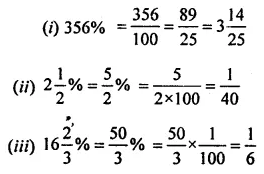Question 2.
Express the following fractions as percentages: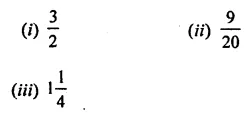Solution: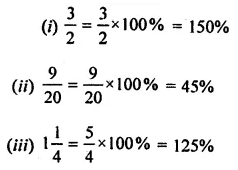Question 3.
Express the following fractions as decimals. Then express the decimals as percentages: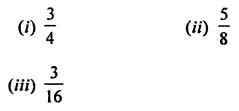Solution: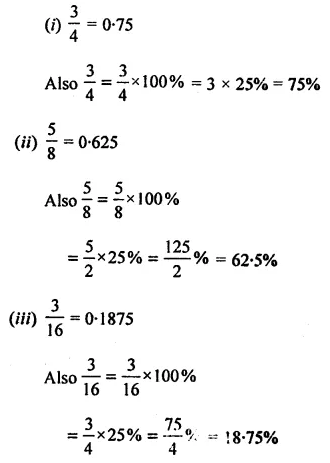Question 4.
Express the following fractions as decimals correct to four decimal places. Then express the decimals as percentages:Solution: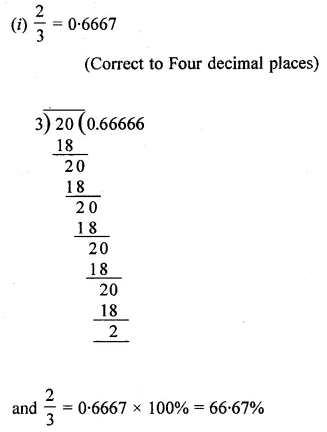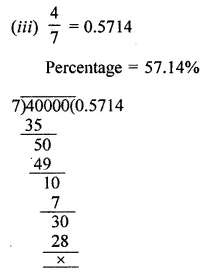Question 5.
Express the following ratios as percentages:
(i) 17 : 20
(ii) 13 : 18
(iii) 93 : 80
Solution:Question 6.
Express the following percentages as decimals:
(i) 20%
(ii) 2%
(iii) $$3 \frac{1}{4}$$%
Solution:Question 7.
Find the value of:
(i) 27% of ₹50
(ii) $$6 \frac{1}{4}$$% of 25 kg
Solution: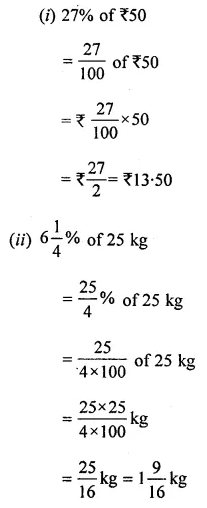Question 8.
What per cent is :
(i) 300 g of 2 kg
(ii) ₹7·50 of ₹6
Solution:Question 9.
What per cent of:
(i) 50 kg is 65 kg
(ii) ₹9 is ₹4
Solution: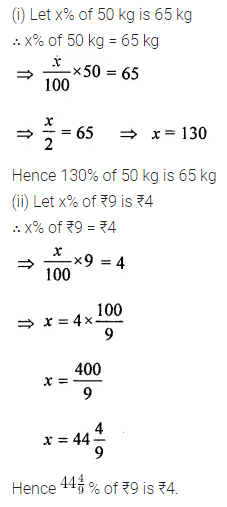Question 10.
(i) If $$16 \frac{2}{3}$$% of a number is 25, find the number.
(ii) If 13·25% of a number is 159, find the number.
Solution: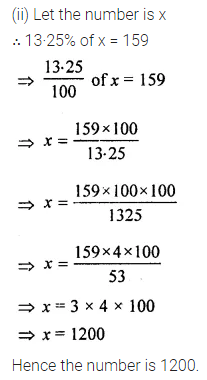Question 11.
(i) Increase the number 60 by 30%
(ii) Decrease the number 750 by 10%
Solution:Question 12.
(i) What number when increased by 15% becomes 299?
(ii) On decreasing the number by 18%, it becomes 697. Find the number.
Solution: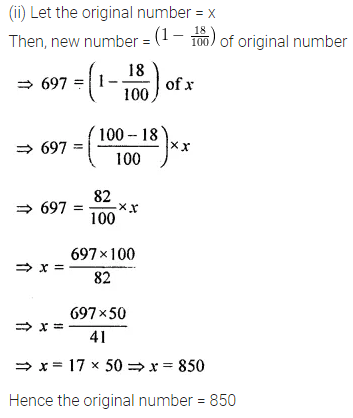Question 13.
Mr Khanna spent 83% of his salary and saved ₹1870. Calculate his monthly salary.
Solution: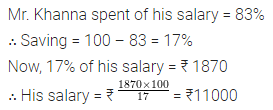Question 14.
In school, 38% of the students are girls. If the number of boys is 1023, find the total strength of the school.
Solution: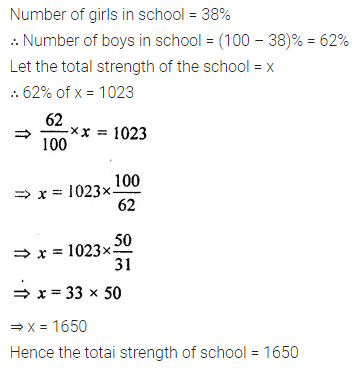Question 15.
The price of an article increases from ₹960 to ₹1080. Find the percentage increase in the price.
Solution: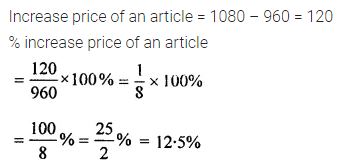Question 16.
In a straight contest, the loser polled 42% votes and lost by 14400 votes. Find the total number of votes polled. If the total number of eligible voters was 1 lakh, find what percentage of voters did not vote.
Solution: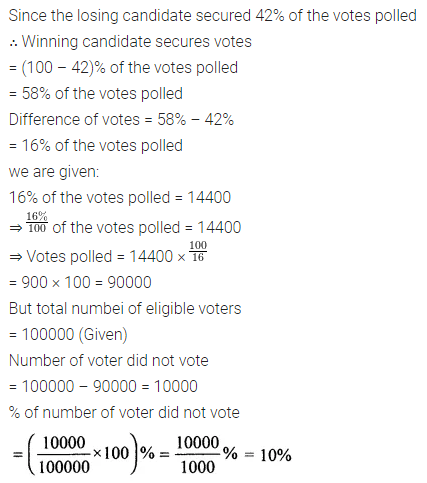Question 17.
Out of 8000 candidates, 60% were boys. If 80% of the boys and 90% of the girls passed the exam, find the number of candidates who failed.
Solution: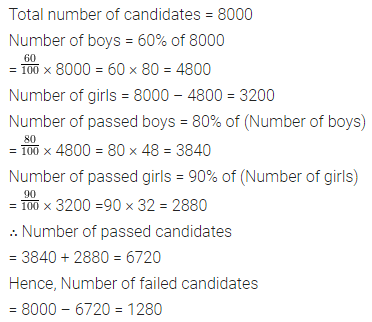Question 18.
In an exam, $$\frac{1}{4}$$ of the students failed both in English and Maths, 35% of students failed in Maths and 30% failed in English.
(i) Find the percentage of students who failed in any of the subjects.
(ii) Find the percentage of students who passed in both subjects.
(iii) If the number of students who failed only in English was 25, find the total number of students.
Solution:Question 19.
On increasing the price of an article by 16%, it becomes ₹1479. What was its original price?
Solution:Question 20.
Pratibha reduced her weight by 15%. If now she weighs 59.5 kg, what was her earlier weight?
Solution: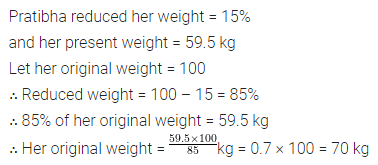Question 21.
In a sale, a shop reduces all its prices by 15%. Calculate:
(i) the cost of an article which was originally priced at ₹40.
(ii) the original price of an article which was sold for ₹20.40.
Solution: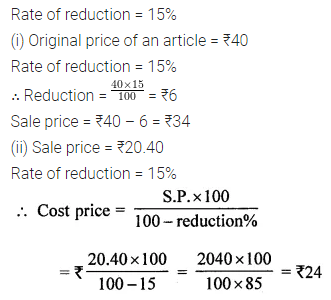Question 22.
Increase the price of ₹200 by 10% and then decrease the new price by 10%. Is the final price same as the original one ?
Solution: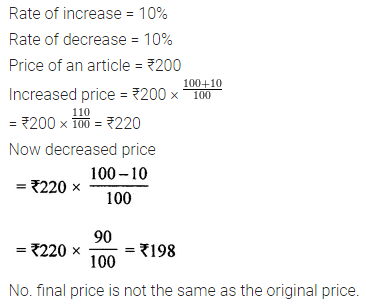Question 23.
Chandani purchased some parrots. 20% flew away and 5% died. Of the remaining, 45% were sold. Now 33 parrots remain. How many parrots had Chandani purchased?
Solution: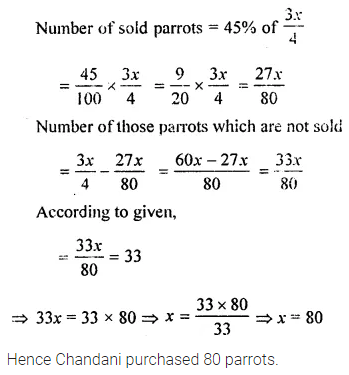Question 24.
A candidate who gets 36% marks in an examination fails by 24 marks but another candidate, who gets 43% marks, gets 18 more marks than the minimum pass marks. Find the maximum marks and the percentage of pass marks.
Solution: